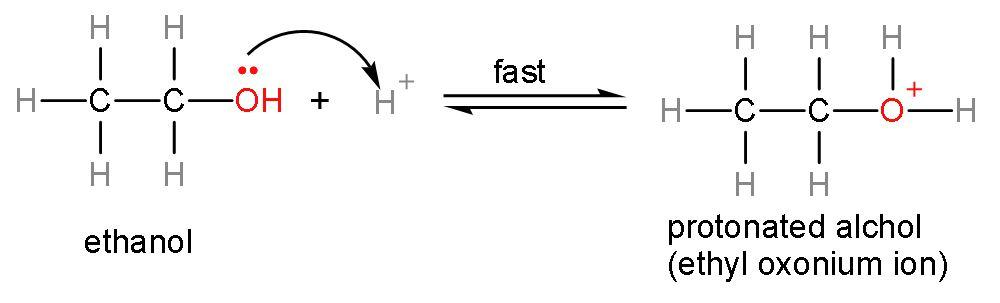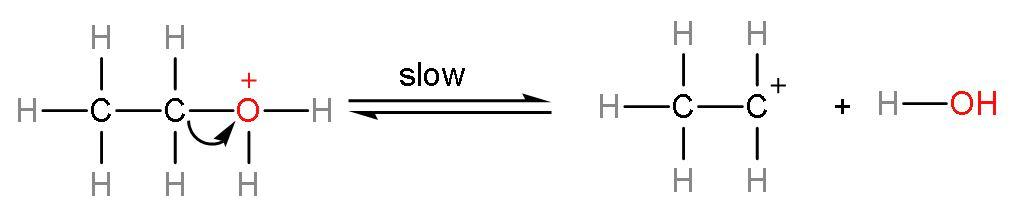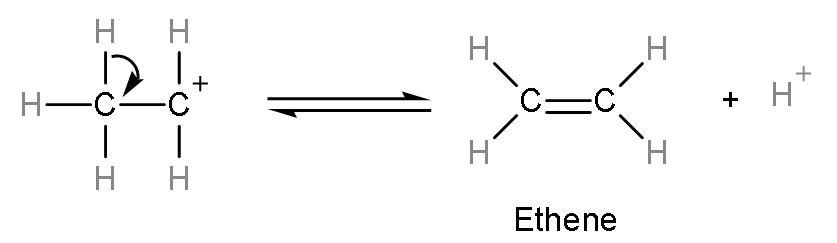QuestionAnswers

# The reaction of ethanol with conc. ${{\text{H}}_{2}}\text{S}{{\text{O}}_{4}}$gives: A. ethaneB. etheneC. ethyneD. ethanoic acid

Hint: The reaction between ethanol and conc. ${{\text{H}}_{2}}\text{S}{{\text{O}}_{4}}$ is a dehydration reaction. Dehydration means removal of water molecules to form alkenes. As concentrated ${{\text{H}}_{2}}\text{S}{{\text{O}}_{4}}$ is a dehydrating agent. Ethanol has two carbon atoms and an alcohol group and rest vacancies are filled by hydrogen atoms.

Let us form the product of this reaction using the mechanism:
Step (1)- Attack of ${{\text{H}}^{+}}$ ion on the lone pair of oxygen atom, as the ${{\text{H}}^{+}}$ ion is electron deficient and lone pairs are electron rich. The ${{\text{H}}^{+}}$ ion is formed from dissociation of sulphuric acid into its respective ions $\left( \text{2}{{\text{H}}^{+}}+\text{SO}_{4}^{2-} \right)$. This attack on lone pairs leads to formation of protonated alcohol.Step (2)- Removal of water occurs from the protonated alcohol to form carbocation. This is a slow step or rate-determining step. The rate of any reaction is determined by the slowest step.Step (3)- Formation of alkene or ethene takes place by the elimination of ${{\text{H}}^{+}}$ion from the carbocation. The ${{\text{H}}^{+}}$ion is a catalyst of this reaction as it remains unutilized.The correct answer to this question is ethene is formed as a product on reaction between ethanol and conc. ${{\text{H}}_{2}}\text{S}{{\text{O}}_{4}}$ So, the correct answer is “Option B”.

Secondary and tertiary alcohols dehydrate under mild conditions only because after protonation, the carbocation formed is highly stable due to hyperconjugation. Thus, the relative ease of dehydration of alcohols is $\text{Tertiary}>\text{Secondary}>\text{Primary}$.
Note: Alcohols reacts with conc.${{\text{H}}_{2}}\text{S}{{\text{O}}_{4}}$ not only to form alkenes but at different temperatures, the reactions are different thus, the products formed are different. Like,
${{\text{C}}_{2}}{{\text{H}}_{5}}\text{OH}+\text{conc}\text{.}{{\text{H}}_{2}}\text{S}{{\text{O}}_{4}}\xrightarrow{383\text{K}}{{\text{C}}_{2}}{{\text{H}}_{5}}\text{HS}{{\text{O}}_{4}}+{{\text{H}}_{2}}\text{O}$
${{\text{C}}_{2}}{{\text{H}}_{5}}\text{OH}+\text{conc}\text{.}{{\text{H}}_{2}}\text{S}{{\text{O}}_{4}}\xrightarrow{413\text{K}}{{\text{C}}_{2}}{{\text{H}}_{5}}{\mathrm O}{{\text{C}}_{2}}{{\text{H}}_{5}}+{{\eta }_{2}}{\mathrm O}$
${{\text{C}}_{2}}{{\text{H}}_{5}}\text{OH}+\text{conc}\text{.}{{\text{H}}_{2}}\text{S}{{\text{O}}_{4}}\xrightarrow{443\text{K}}{{\text{C}}_{2}}{{\text{H}}_{4}}+{{\eta }_{2}}{\mathrm O}$